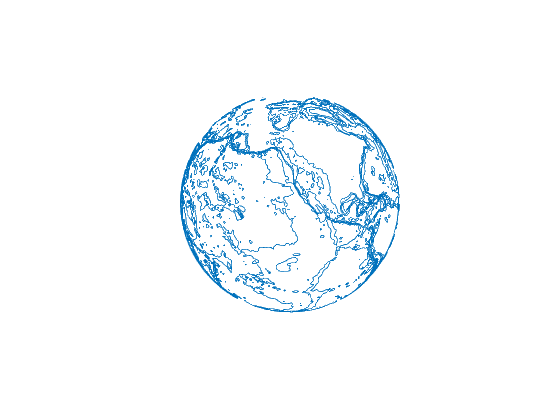# globecontour documentation

The globecontour function plots contour lines on a globe from gridded data. Note: these contours are not contour graphics objects and are not linked to the current colormap.

## Syntax

```globecontour(lat,lon,Z)
globecontour(lat,lon,Z,n)
globecontour(lat,lon,Z,v)
globecontour(...,PropertyName,PropertyValue)
h = globecontour(...)```

## Description

globecontour(lat,lon,Z) plots contour lines for the georeferenced data in Z on a globe with radius defined as 6371, where 6371 corresponds to the average radius of the Earth in kilometers. The inputs lat and lon are the same size as Z and can be defined for arbitrary domains using the meshgrid function.

globecontour(lat,lon,Z,n) plots n equally-spaced contour lines corresponding to the georeferenced data in Z.

globecontour(lat,lon,Z,v) plots contour lines at heights specified by the vector v.

globecontour(...,PropertyName,PropertyValue) specifies the line properties to control contour line appearance and behavior.

h = globecontour(...) returns the handle h of the plotted objects.

## Example 1: Topographic contours

Start by using cdtgrid, and topo_interp to get global topography:

```[lat,lon] = cdtgrid;
topo = topo_interp(lat,lon);
```

Now plot 10 contour lines depicting Earth's topography:

```figure
globecontour(lat,lon,topo,10)
```Set the globe color to white using globefill:

```hold on
globefill
axis tight
```Add black contour lines depicting the topography Earth's oceans from 7000 m below sea level to sea level using 500 m spacing:

```globecontour(lat,lon,topo,-7000:500:0,'color','k')
```Add orange contour lines depicting the topography Earth's landmass from sea level to 5500 m above sea level using 500 m spacing:

```globecontour(lat,lon,topo,0:500:5500,'color',rgb('orange'))
```Plot sea level (the coastline) as a thick green line:

```globecontour(lat,lon,topo,[0 0],'color',rgb('green'),'linewidth',3)
view([30 30])
```## Example 2: Surface pressure

For this example, plot the global surface pressure anomaly for May 2017. First, load the data:

```filename = 'ERA_Interim_2017.nc';

% Grid the lat,lon arrays:
[Lat,Lon] = meshgrid(lat,lon);

% Calculate the May surface pressure anomaly:
spa = sp(:,:,5) - mean(sp,3);
```

Now plot the surface pressure anomaly as 30 contours on top of a Blue Marble globe plot:

```figure
globeimage
globecontour(Lat,Lon,spa,30)
view(45,20)
axis tight
```## Author Info

This function and supporting documentation were written by Natalie S. Wolfenbarger for the Climate Data Toolbox for Matlab, 2019.